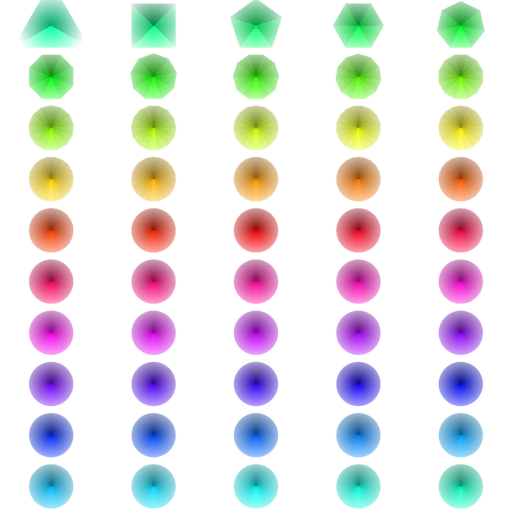# Continuously discrete

## November 5, 2012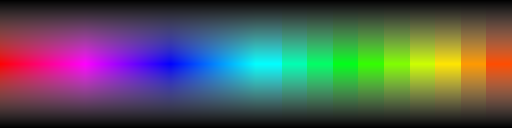Smooth looking images have every pixel quite similar to the ones all around them, while still being different enough to form a picture that isn't just one solid colour.

When a shader program is used to make an image, it is typically fed with input values (say x and y coordinates) that are only slightly different for each pixel.

If those slight differences are preserved in the calculations the shader makes, the output image will be smooth.

Interesting images however need changes in them. Tools like step, mod and abs are good for creating those, but still they tend to generate quite predictable images, which are actually not so interesting.

What we could really make use of is a way to create <i>discrete</i> values from continuous values. That would let us make different parts of an image clearly different.

Say I want to make a very simple picture - four coloured squares, each one a different colour. Let's try with step first:

```vec2 s=step(0.,c.xy);
f=vec4(s.x,s.y,0.,1.)
```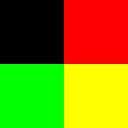Applying the step to x and y makes 4 regions around the center (0,0) point. Negative values are zero, positive values are 1. Mapping x and y to red and green gives us four colours - 0+0=black,0+1=green,1+0=red,1+1=yellow.

Can the same approach now gives us 9 different coloured squares?

```vec2 s=0.5*(step(-0.333,c.xy)+step(0.333,c.xy));
f=vec4(s.x,s.y,0.,1.)
```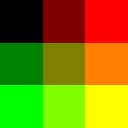Well, yes. Adding the result of 2 steps at minus and plus 1/3rd and scaling the result to be between 0 and 1, now with intermediate values of 1/3 and 2/3, worked. But it's clear this approach won't scale up if we want 10 or 100 steps.

So, what would be another approach? If we would scale up our input range to the number of steps we want, in this case 0-3, and then simply throw away the fractional parts, so that 1.2 becomes 1, and 2.3 becomes 2, and finally scale the result back, that should give us the same result.

The GLSL function we need here is floor. Let's try it out:

```vec2 s=floor(3.*(c.xy*.5+.5))/2.;
f=vec4(s.x,s.y,0.,1.)
```The looks practically identical to the double step version, but now we only have one function. The coordinate calculation was a bit messy because we had to scale from -1-1 to 0-3, then we divided by 2 to get the result back in the range 0-2.

(You may have spotted that at the right edge the result is 3, not 2, which scales back down to 1.5 instead of 1. But it doesn't matter in this case because that still clamps to 1 when used as a colour value).

The big advantage of our new approach is that it scales to any number of steps. Here's a 5x10 grid which is almost the same as the 3x3. But this time, instead of a gradient, I'm going to use it to count to 50. In shades of grey:

```vec2 s=floor(vec2(5.,10.)*(c.xy*.5+.5));
f=vec4(0.02*(s.x+5.*s.y))
```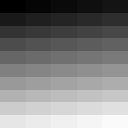Using our new-found ability to count in a shader, why don't we combine that with something we developed earlier. Here's 50 shapes. All grey, of course.

```vec2 r=vec2(5.,10.)*(c.xy*.5+.5);
vec2 i=floor(r);
vec2 c=(fract(r)-.5)*vec2(4.,2.)*.6;
float s=3.+i.x+5.*i.y;
float a=atan(c.x,c.y);
float b=6.28319/s;
float w=floor(.5+a/b);
float g=smoothstep(.55,.5,cos(w*b-a)*length(c.xy));
f=vec4(vec3(1.-g*((60.-s)/50.)),g);
```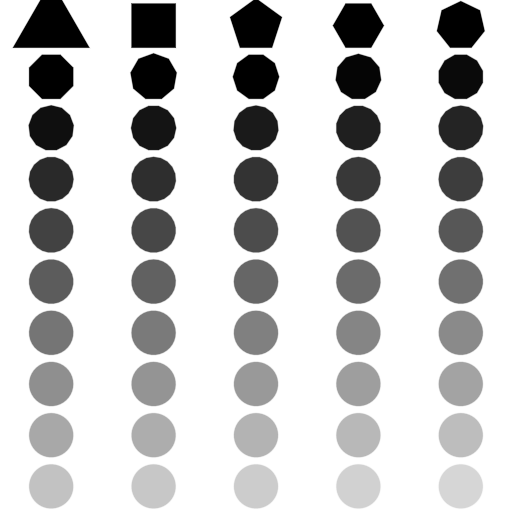The polygon shader from earlier took a parameter for the number of sides. By feeding the counting value to the polygon shader, we get polygons with increasing numbers of sides depending on the count value in that region of the image. It also uses the count to choose the grey shade.

But I think I've had enough of grey shades for now, and I've also had enough of RGB.

A different model for mixing colours is HSV - Hue (colour), Saturation (whiteness) and Value (darkness). A nice feature of hue is that it repeats - a hue of 1 is the same as a hue of 0.

I'm going to finish now by using our count to pick a hue, the polygon side to pick a value, and the distance from the centre to pick a saturation.

That should make, well, something.

```vec2 r=vec2(5.,10.)*(c.xy*.5+.5);
vec2 i=floor(r);
vec2 c=(fract(r)-.5)*vec2(4.,2.)*.6;
float s=3.+i.x+5.*i.y;
float a=atan(c.x,c.y);
float b=6.28319/s;
float w=floor(.5+a/b);
float g=smoothstep(.55,.5,cos(w*b-a)*length(c.xy));
float v=1.-1.2*length(c.xy);
float sa=1.-abs(w/s);
float h=s/50.;
vec3 rgb=v*sa*clamp(abs(mod(h*6.+vec3(0.,2.,4.),6.)-3.)-1.,0.,1.)+(v-v*sa);
f=vec4(1.-g*rgb,g);
```